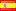# Revision history [back]

### Translating a list of polynomials using sage

I have a list of polynomials in Sage as follows:

n=5
W=[P.coxeter_polynomial() for P in posets(n)]


Now I want to have the list of all such polynomials as polynomials in R.<x> = PolynomialRing(ComplexField(100)) . Is there an easy way to translate the above list W so that Sage views every polynomial in that list as a polynomial in R.<x> = PolynomialRing(ComplexField(100)).

### Translating a list of polynomials using sage

I have a list of polynomials in Sage as follows:

n=5
W=[P.coxeter_polynomial() for P in posets(n)]


Now I want to have the list of all such polynomials as polynomials in R.<x> = PolynomialRing(ComplexField(100)) . .

My question is: Is there an easy way to translate the above list W so that Sage views every polynomial in that list as a polynomial in R.<x> = PolynomialRing(ComplexField(100)).PolynomialRing(ComplexField(100))?3 NoneJuanjo2733 ●2 ●33 ●71

### Translating a list of polynomials using sage

I have a list of polynomials in Sage as follows:

n=5
W=[P.coxeter_polynomial() for P in posets(n)]


Now I want to have the list of all such polynomials as polynomials in R.<x> = PolynomialRing(ComplexField(100)) PolynomialRing(ComplexField(100)) .

My question is: Is there an easy way to translate the above list W so that Sage views every polynomial in that list as a polynomial in R.<x> = PolynomialRing(ComplexField(100))?PolynomialRing(ComplexField(100))?